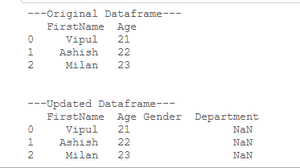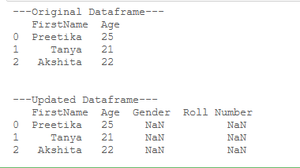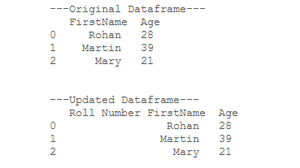# How to add Empty Column to Dataframe in Pandas?

In data Frames, Empty columns are defined and represented with NaN Value(Not a Number value or undefined or unrepresentable value). There are various methods to add Empty Column to Pandas Dataframe.

Method 1: Using the Assignment Operator.

This method is used to forcefully assign any column a null or NaN value.

## Python3

 `# import required libraries ` `import` `numpy as np ` `import` `pandas as pd ` ` `  `# create a Dataframe ` `Mydataframe ``=` `pd.DataFrame({``'FirstName'``: [``'Vipul'``, ` `                                          ``'Ashish'``, ` `                                          ``'Milan'``], ` `                            ``"Age"``: [``21``,``22``,``23``]}) ` `# show the dataframe ` `print``(``"\n\n---Original Dataframe---\n"``,  ` `      ``Mydataframe) ` ` `  `# add an empty columns ` `Mydataframe[``'Gender'``] ``=` `'' ` `Mydataframe[``'Department'``] ``=` `np.nan ` ` `  `# show the dataframe ` `print``(``"---Updated Dataframe---\n"``,  ` `      ``Mydataframe)`

Output:In the above example, we are using the assignment operator to assign empty string and Null value to two newly created columns as “Gender” and “Department” respectively for pandas data frames (table). Numpy library is used to import NaN value and use its functionality.

Method 2: Using Dataframe.reindex().

This method is used to create new columns in a dataframe and assign value to these columns(if not assigned, null will be assigned automatically).
Example :

## Python3

 `# import pandas library ` `import` `pandas as pd ` ` `  `# create a dataframe ` `Mydataframe ``=` `pd.DataFrame({``'FirstName'``: [``'Preetika'``, ` `                                          ``'Tanya'``, ` `                                          ``'Akshita'``], ` `                            ``"Age"``: [``25``,``21``,``22``]}) ` `# show the dataframe ` `print``(``"---Original Dataframe---\n"``, ` `      ``Mydataframe) ` ` `  `# add an empty columns ` `Mydataframe ``=` `Mydataframe.reindex(columns ``=` `Mydataframe.columns.tolist()  ` `                                  ``+` `[``'Gender'``,``'Roll Number'``]) ` ` `  `# show the dataframe ` `print``(``"\n\n---Updated Dataframe---\n"``, ` `      ``Mydataframe) `

Output:In the above example, we created a data frame with two columns “First name and “Age” and later used Dataframe.reindex() method to add two new columns “Gender” and ” Roll Number” to the list of columns with NaN values.

Method 3: Using Dataframe.insert().

This method is used to add a new column to a pandas dataframe at any index location we want and assign the appropriate value as per need.
Example:

## Python3

 `# import pandas library ` `import` `pandas as pd ` ` `  `# create a dataframe ` `Mydataframe ``=` `pd.DataFrame({``'FirstName'``: [``'Rohan'``, ` `                                          ``'Martin'``, ` `                                          ``'Mary'``], ` `                            ``"Age"``: [``28``,``39``,``21``]}) ` `# show the dataframe ` `print``(``"---Original Dataframe---\n"``, ` `      ``Mydataframe) ` ` `  `# add an empty column ` `Mydataframe.insert(``0``,``'Roll Number'``,'') ` ` `  `# show the dataframe ` `print``(``"\n\n---Updated Dataframe---\n"``, ` `      ``Mydataframe) `

Output:In the above example, we are using the Dataframe.insert() method on pandas data frames (table) to add an empty column “Roll Number”, here we can also insert the column at any index position we want (as here we placed the value at index location 0).

My Personal Notes arrow_drop_upCheck out this Author's contributed articles.

If you like GeeksforGeeks and would like to contribute, you can also write an article using contribute.geeksforgeeks.org or mail your article to contribute@geeksforgeeks.org. See your article appearing on the GeeksforGeeks main page and help other Geeks.

Please Improve this article if you find anything incorrect by clicking on the "Improve Article" button below.

Article Tags :

Be the First to upvote.

Please write to us at contribute@geeksforgeeks.org to report any issue with the above content.